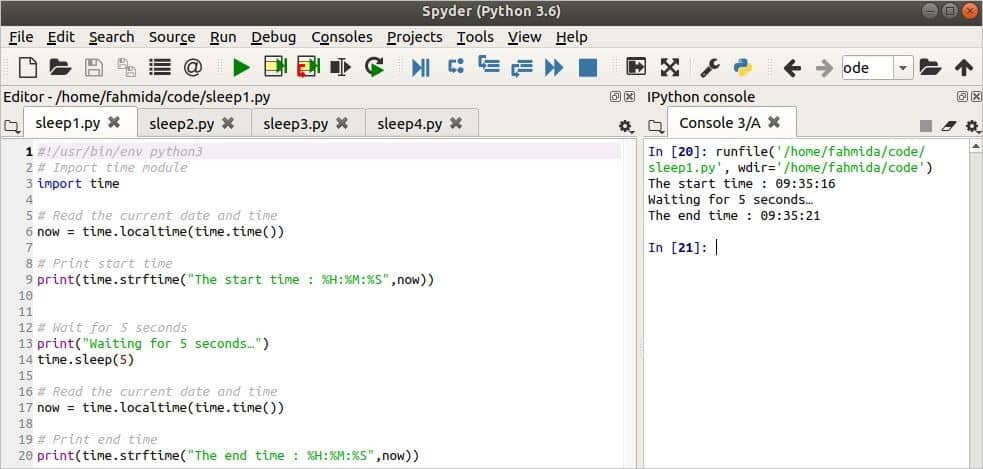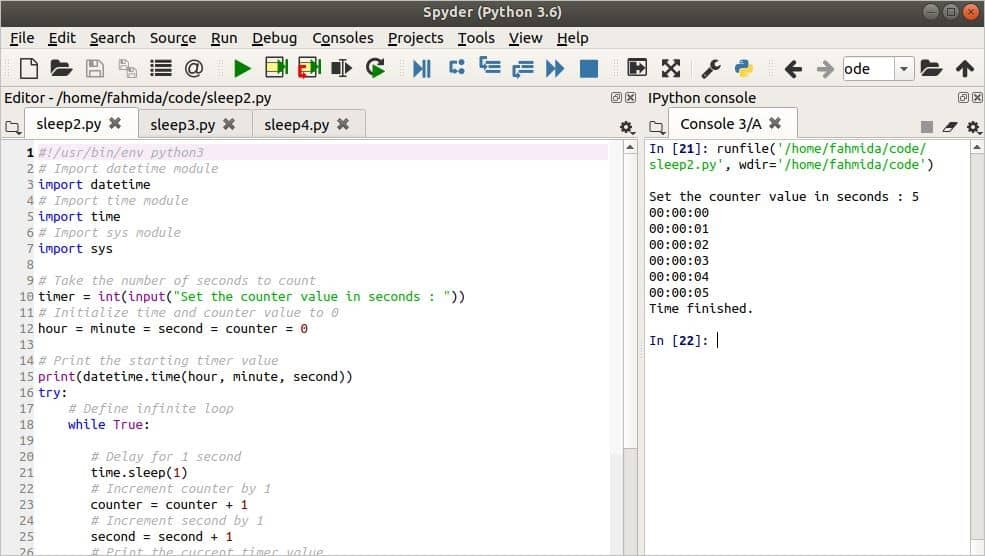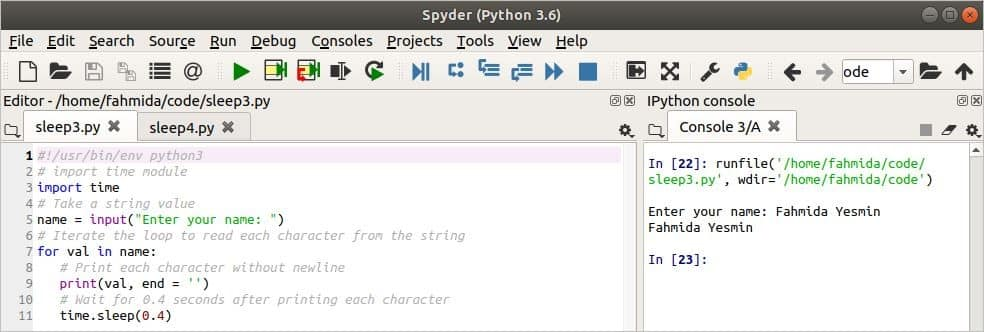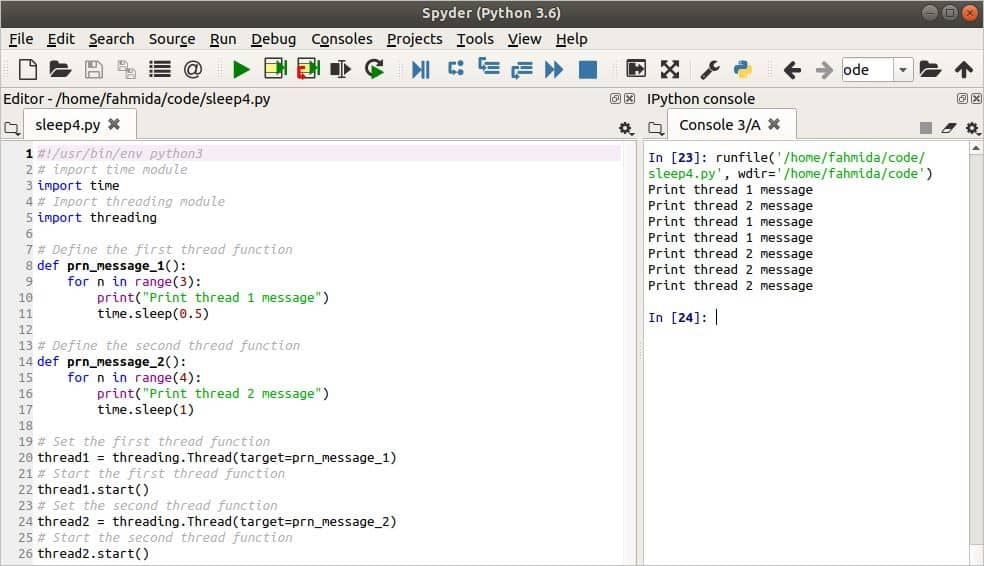Python

# Using the Python sleep() Method

If you want to set a time delay before executing any script, then you can use the sleep() function that is built into Python. This method exists under the time module. Generally, the sleep() function is used to halt the execution of the program for the given time period (in seconds). For example, if you are downloading a file from a location using a script that may require a certain time period, then you can use the sleep() method to inform the user to wait. This tutorial will show you some of the different uses of the sleep() function in Python 3.

### Syntax

The following example shows the simple use of the sleep() function to halt program execution in the middle of the script for a certain period. The script will read and print the current system time value, wait for 5 seconds under the sleep() function, and again read and print the current system time. Here, localtime() and time() functions are used for reading the current system date and time, and the strftime() function is used to print the formatted time.

#!/usr/bin/env python3
# Import time module
import time

# Read the current date and time
now = time.localtime(time.time())

# Print start time
print(time.strftime("The start time : %H:%M:%S",now))

# Wait for 5 seconds
print("Waiting for 5 seconds…")
time.sleep(5)

# Read the current date and time
now = time.localtime(time.time())

# Print end time
print(time.strftime("The end time : %H:%M:%S",now))

Output

The following output will appear after running the above script. The output shows that the duration between the start time and the end time is 5 seconds, using the sleep() function.### Example 2: Create A Time Counter Using sleep()

The following example shows how to easily create a time counter via the sleep() method. The script will take a number in seconds to initialize the time counter variable.  Three modules are imported here. The datetime module is imported to set the initial time value. The time module is imported to use the sleep() function. The sys module is used to terminate the infinite while loop when the counter value equals to the time counter value. Four variables are used in the script to initialize the hour, minute, second, and counter variables to set the starting time of the time counter. Here, the sleep() function will wait for one second in each iteration of the loop and then print the time counter value.

#!/usr/bin/env python3
# Import datetime module
import datetime
# Import time module
import time
# Import sys module
import sys

# Take the number of seconds to count
timer = int(input("Set the counter value in seconds : "))
# Initialize time and counter value to 0
hour = minute = second = counter = 0

# Print the starting timer value
print(datetime.time(hour, minute, second))
try:
# Define infinite loop
while True:

# Delay for 1 second
time.sleep(1)
# Increment counter by 1
counter = counter + 1
# Increment second by 1
second = second + 1
# Print the current timer value
print(datetime.time(hour, minute, second))
# Set the second value to 0 when the value is more than 60
if second > 60:
minute = minute + 1
second = 0
# Set the minute value to 0 when the value is more than 60
if minute > 60:
hour = hour + 1
minute = 0
# Terminate the loop when the counter value is equal to timer value
if counter == timer:
print("Time finished.")
break

except KeyboardInterrupt:

# Terminate the script when Ctrl+C is pressed
sys.exit

Output

The following output will appear after running the script.### Example 3: Print Each Character of A String with A Delay

If you want to display each character of a text with some delay in the output, then you can apply the sleep() function in the script. A similar task is shown in the following script. Here, a text value is taken as the input. Each character of the text is iterated using the loop and the function will wait for 0.4 seconds before printing.

#!/usr/bin/env python3
# import time module
import time

# Take a string value
name = input("Enter your name: ")
# Iterate the loop to read each character from the string
for val in name:
# Print each character without newline
print(val, end = '')
# Wait for 0.4 seconds after printing each character
time.sleep(0.4)

Output

Run the script. You will understand the output properly after running the script.### Example 4: Use of sleep() in Multi-Threaded Script

The following example shows the use of the sleep() function in the multi-threaded Python script. Two modules are imported here: the time module for using the sleep() function and the threading module for creating multiple threads. Two custom functions are used in the script. A text message will print and wait for 0.5 seconds for three times inside the for loop when the first thread starts. A text message will print and wait for 1 second for four times inside the for loop when the second thread starts.

#!/usr/bin/env python3
# import time module
import time

# Define the first thread function
def prn_message_1():
for n in range(3):
time.sleep(0.5)

# Define the second thread function
def prn_message_2():
for n in range(4):
time.sleep(1)

# Set the first thread function
# Start the first thread function
# Set the second thread function
# Start the second thread function

Output

The following output will appear after running the script. After starting the first thread, the second thread begins and wait until completion for 1 second. During this time, the loop of the first thread is completed the last two iterations. The last three outputs have appeared for the loop of the second thread.### Conclusion

The sleep() function is used to solve many different types of time-related problems in Python. Various uses of this method are explained by using very easy examples here to help the reader know the functions of this tool. I believe that you or any other Python user will be able to use this method properly after practicing the examples included in this tutorial.